# ISEE Middle Level Quantitative : How to find the part from the whole

## Example Questions

### Example Question #1 : Whole And Part

Harvey bought a suit at a 25% employee discount at the store where he works. The suit originally cost $350.00. How much did he end up paying? Possible Answers: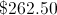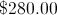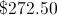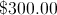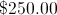Correct answer:Explanation: Finding 25% of a number is the same as multiplying it by 0.25; to get the discount, multiply 0.25 by the original purchase price of$350.00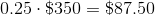To get the price Harvey paid, subtract the discount from the original price: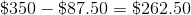### Example Question #2 : Whole And Part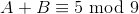Which of the following is true if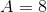?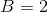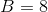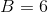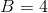Explanation:

Two expressions are equivalent in modulo 9 arithmetic if and only if, when each is divided by 9, the same remainder is yielded.,

so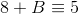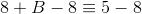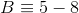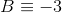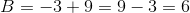, sois the correct choice.

### Example Question #3 : Whole And Partis a positive integer. Which is the greater quantity?

(a) The remainder if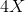is divided by 5

(b) The remainder if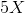is divided by 4

(a) and (b) are equal

(b) is the greater quantity

It is impossible to determine which is greater from the information given

(a) is the greater quantity

It is impossible to determine which is greater from the information given

Explanation:

The information is insufficient.

For example, if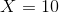: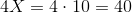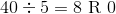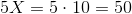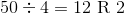This gives the division in (b) the greater remainder.

But if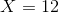: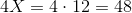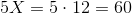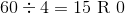This gives the division in (a) the greater remainder.

### Example Question #1 : Whole And Partis a positive even integer. Which is the greater quantity?

(a) The remainder if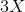is divided by 6

(b) The remainder if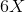is divided by 3.

(a) and (b) are equal

(a) is the greater quantity

It is impossible to determine which is greater from the information given

(b) is the greater quantity

(a) and (b) are equal

Explanation:

Sinceis an even integer, by definition, there is an integer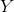such that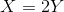.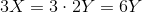; therefore,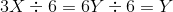; the remainder is 0.

Also,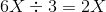; the remainder is 0.

The two remainders are both equal to 0.

### Example Question #5 : Whole And Partis a positive odd integer. Which is the greater quantity?

(a) The remainder ifis divided by 8

(b) The remainder if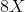is divided by 4

(a) and (b) are equal

(b) is the greater quantity

(a) is the greater quantity

It is impossible to determine which is greater from the information given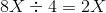, with a remainder of 0.
If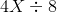were to yield a remainder of 0, then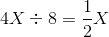must be a whole number; this can only happen ifis even. Sinceis odd, it follows that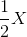is not a whole number, andmust yield a nonzero remainder. (a) must be the greater quantity.